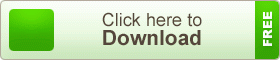# SSC Higher Mathematics Note 9th Chapter Exponential & Logarithmic Functions

SSC Higher Mathematics Note 9th Chapter Exponential & Logarithmic Functions. Exponential functions occur in many contexts, like population growth, compound interest. With the advent of calculations, use of tables of logarithms and antilogarithms has become redundant. But exponential and logarithmic functions retain their place and importance in the theory and applications of mathematics.

## SSC Higher Mathematics Note 9th Chapter Exponential & Logarithmic FunctionsWe discuss the trigonometrical ratios of an acute angle of this chapter. By the ratios of an acute angle, we can determine the technique of any trigonometrical angle. The relation among the ratios and its sign in the different quadrant can be explained. Some identity about trigonometry ratios also is conceptualized.

teachingbd24.com is such a website where you will get all kinds of necessary information regarding educational notes, suggestions and question patterns of schools, colleges, and madrasas. Particularly, you will get here special notes of physics that will be immensely useful to both students and teachers. The builder of the website is Mr. Md. Shah Jamal who has been serving for 33 years as an Assistant Professor of Physics at BAF Shaheen College Dhaka. He expects that this website will meet up all the needs of Bengali version learners /students. He has requested concerned students and teachers to spread this website home and abroad.

SSC Higher Mathematics Note